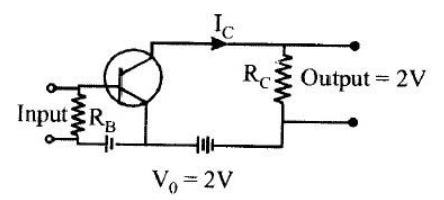QuestionAnswers

# In a CE transistor amplifier, the audio signal voltage across the collector resistance of $2k\Omega$ is $2V$. If the base resistance is $1k\Omega$ and the current amplification of the transistor is $100$, the input signal voltage isA) $2mV$B) $3mV$C) $10mV$D) $0.1mV$Verified
130.8k+ views
Hint: Use the formula of parameter $\beta$ $\left( {\beta= \dfrac{{{I_C}}}{{{I_B}}}} \right)$ for current gain. In common-emitter configuration of a NPN transistor, the input current ${I_B}$ is through the base junction, and the collector current ${I_C}$ is the output.

Complete step by step solution:Here, voltage across the collector ${V_0} = 2V$
Base resistance ${R_B} = 1 \times {10^3}\Omega$
Collector resistance ${R_C} = 2 \times {10^3}\Omega$
And we have to find the input signal voltage.
The common emitter (CE) amplifier is used when the large current gain is needed. The input signal is applied between the base and emitter terminals whereas the output signal is taken between the collector and emitter terminals.
So we know, the voltage across the collector is
${V_0} = 2V$
Or ${V_0} =$(collector current) $\times$(collector resistance)
Let the collector current be ${I_C}$
So, ${V_0} = {I_C}{R_C} = 2$
Since ${R_C} = 2 \times {10^3}$, therefore we calculate collector current as follows
${I_C} = \dfrac{{{V_0}}}{{{R_C}}} \\ \Rightarrow {I_C} = \dfrac{2}{{2 \times {{10}^3}}} = {10^{ - 3}} \\$
Now, $\beta$ is the current gain or current amplification factor.
So $\beta= \dfrac{{{I_C}}}{{{I_B}}}$
Here, ${I_C} = {10^{ - 3}}$and $\beta= 100$
Therefore, to find base current as
${I_B} = \dfrac{{{I_C}}}{\beta } \\ \Rightarrow {I_B} = \dfrac{{{{10}^{ - 3}}}}{{100}} = {10^{ - 5}}A \\$
Now let the input signal voltage is ${V_i}$
Since the base is input terminal, therefore
${V_i} =$(Base resistance) $\times$ (Base current)
$\Rightarrow {V_i} = {R_B}{I_B}$
By putting the value of ${R_B}$and${I_B}$, we get
${V_i} = \left( {1 \times {{10}^3}} \right) \times \left( {{{10}^{ - 5}}} \right) \\ \Rightarrow {V_i} = {10^{3 - 5}} = {10^{ - 2}} = 0.1mV \\$

$\therefore$ The input signal voltage is $0.1mV$. Hence the correct option is (D).

Note:
The interesting thing to know about the CE transistor amplifier is that the emitter terminal is common for both input and output signals that’s why it is called a common emitter transistor. Moreover, the current gain of a transistor in CE configuration is high. Therefore, the transistor in CE configuration is used for amplifying the current.